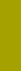﻿ Grain sizeSand Texture Sedimentology | Variables

Grain size

Variable 2: Logarithmic Grain Size, PHI

Synonym of ‘grain’: particle

Because it can be directly measured, grain size is the natural variable of sieving. Surprisingly, sedimentation is capable of calculating most accurate grain size, with unprecedented accuracy and resolution. Such a grain size should be indicated as sedimentational grain size. Because sedimentation of sand sized particles is resisted in proportion to specific surface, and the specific surface is proportional to both the particle fineness and nonsphericity, the sedimentational grain size, in constrast to sieve size, is consistent with specific surface (nonsphericity is inversely proportional to sieve size because it causes an apparent coarsening of the sieved particles). Whereas even the most precise sieving cannot approach sedimentation in accuracy and resolution, sieving can not replace sedimentation and sedimentation cannot replace sieving in all situations. But sedimentation combined with precise sieving can yield considerably enhanced information (see also 'Sedimentation vs. Sieving', page 2).

According to W.C. KRUMBEIN (1934), the grain size is expressed in negative binary logarithm (logarithm to the base 2), PHI (English transcription of the Greek letter f or capital Ø):

PHI = -log2d[mm/mm].

The Macrogranometer’s™ PHI range:

 PHI mm mm the coarse limit -2.00 2+2 4.000 the fine limit +5.00 2-5 0.015625

Our SedVar™ software calculates grain size from settling rate according to the equations (1) through (7) of J. BREZINA (1979), which enable us to include a specified grain shape. Shape Factor SF of Arthur Thomas COREY (1949) and J. S. McNOWN + J. MALAIKA (1950) is used as a hydraulically effective characteristic. It is defined as a dimensionless ratio number relating the minimum, medium and maximum mutually perpendicular particle dimensions, a, b, c respectively, known as the Corey’s Shape Factor (in this paper, the original symbol SFis preferred to “CSF“ used in recent publications of some authors):

SF = a.(b.c)-0.5.

As defined, the maximum value 1.0 is reached if a = b = c. This maximum is valid not only for isometric (naturally worn, irregular) particles but also for smooth spheres. However, physically and in particular hydrodynamically smooth spheres behave much differently than isometric particles having some surface roughness. The grain surface roughness, due to lack of both data and measuring method, could not be treated separately in the equations (1) through (7) of J. BREZINA (1979), and the available data is valid for irregular naturally worn particles. Therefore the less common smooth spheres became the hydrodynamic shape factor (indicated by SF’) greater (SF’ about 1.18) than isometric (rough) particles, such as a cube (SF’ about 1.0). A roughness coefficient, hydrodynamically consistent with the Shape Factor, should be developed and used jointly with it. In this paper, the hydrodynamic Shape Factor (values up to 1.0) is a compound coefficient including basic shape and roughness. Roughness reduction or absence causes an SF’ increase of up to by approximately 18% (for isometric particles up to SF’=1.18).

Because the most typical SF’ values of beach and river sands are approximately SF’=0.65, this value is more suitable than SF’=1.18, which refers to the nearly non-existent (in nature) smooth spheres. For standardization reasons, I recommend SF’=0.65 and Rs (density) = 2.65 g/cm3 for routine usage. Only if both the particle shape and density are fairly constant and specified, can the variable grain size be calculated.

The PSI - PHI conversion implies a change of distribution features (J. BREZINA, 1963). The following four diagrams demonstrate this effect as described below. Fig. 2 shows three normal (=Gaussian) PSI distributions with identical standard deviation: S.D. = 1.0 PSI, but each with a different mean: MEAN = -1 PSI, -2 PSI, -3 PSI. Plotted on a PSI probability chart, these distributions form parallel straight lines. The Y axis is scaled in standard deviation units instead of percentage (see Figure 1). Figures. 3, 4 and 5 show the three PSI distributions from the Fig. 2 converted into PHI distributions with various Shape Factor values: SF = 1.2 through 0.1 by 0.1 step. Note that these PHI distributions are bent toward coarser size (=smaller or more negative PHI values), which means a negative PHI skewness (see Fig. 8). Also, the bending increases the general slope of the PHI distribution curves, which means an increasing (and changing) standard deviation (see also Fig. 7).

In order to minimize the distortions due to shape factor ignorance, Jiri BREZINA (1979) introduced a hydrodynamic equivalence to a rotational ellipsoid specified by a shape factor. This equivalence includes not only the same standard settling rate, particle volume and density, but also the same basic shape; it leads to the hydrodynamic shape factor SF’.